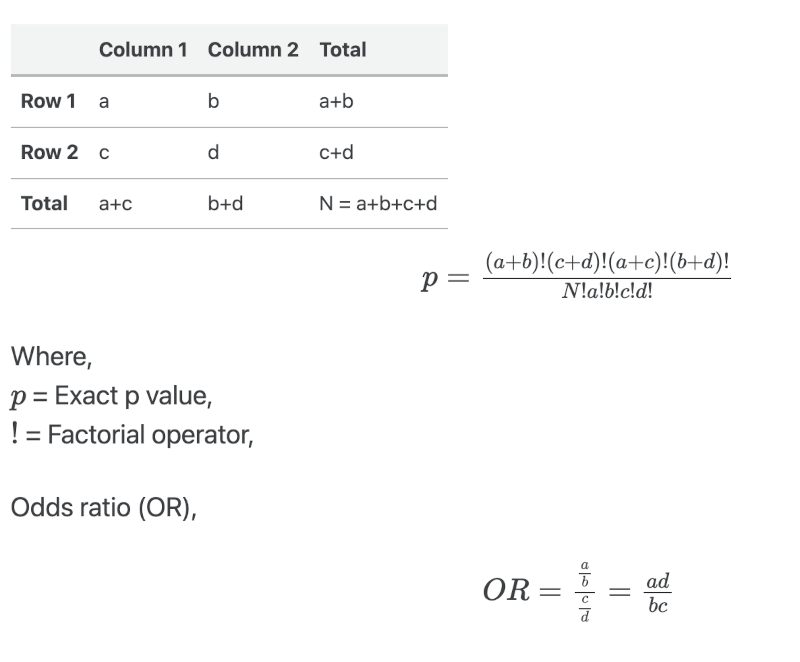# Fisher’s exact test calculator (with formula)

Renesh Bedre    less than 1 minute read

## Fisher’s exact test

Fisher’s exact test is employed to study the association between the two independent categorical variables in a contingency table.

Fisher’s exact test is used when the sample size is small (say, < 1000). The Chi-squared test is appropriate when the sample size is large. Fisher’s exact test is specifically applied when the expected frequencies are less than 5 in more than 20% of cells in a contingency table.

## Fisher’s exact test calculator for 2 x 2 contingency table

• Enter the value ≤ 100 for each cell (a, b, c, and d)
• Odds ratio calculated using sample odds ratio method

## Fisher’s exact test formula

Fisher’s exact test 2 ✕ 2 contingency table is calculated as,If you have any questions, comments, corrections, or recommendations, please email me at reneshbe@gmail.com